Next: Exercises Up: Maxwell's Equations Previous: Electromagnetic Energy Conservation

# Electromagnetic Momentum Conservation

Let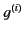be the density of electromagnetic momentum directed parallel to the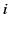th Cartesian axis. (Here,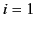corresponds to the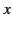-axis,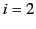to the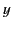-axis, and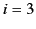to the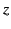-axis.) Furthermore, let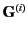be the flux of such momentum. We would expect the conservation equation for electromagnetic momentum directed parallel to theth Cartesian axis to take the form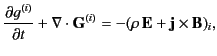(111)

where the subscriptdenotes a component of a vector parallel to theth Cartesian axis. The term on the right-hand side is the rate per unit volume at which electromagnetic fields gain momentum parallel to theth Cartesian axis via interaction with matter. Thus, the term is minus the rate at which matter gains momentum parallel to theth Cartesian axis via interaction with electromagnetic fields. In other words, the term is minus theth component of the force per unit volume exerted on matter by electromagnetic fields. [See Equation (10).] Equation (111) can be generalized to give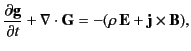(112)

where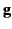is the electromagnetic momentum density (theth Cartesian component ofis thus), and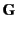is a tensor (see Section 12.5) whose Cartesian components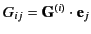, where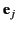is a unit vector parallel to the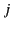th Cartesian axis, specify the flux of electromagnetic momentum parallel to theth Cartesian axis across a plane surface whose normal is parallel to theth Cartesian axis. Let us attempt to derive an expression of the form (112) from Maxwell's equations.

Maxwell's equations are as follows: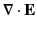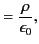(113)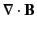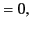(114)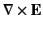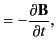(115)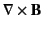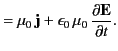(116)

We can take the vector product of Equation (116) divided by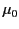with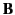, and rearrange, to give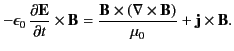(117)

Next, we can take the vector product of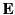with Equation (115) times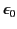, rearrange, and add the result to the previous equation. We obtain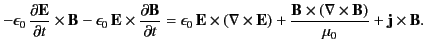(118)

Making use of Equations (113) and (114), we get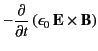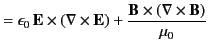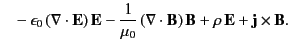(119)

Now,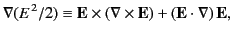(120)

with a similar equation for. Hence, Equation (119) can be written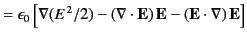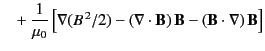(121)

Finally, when written in terms of components, the above equation becomes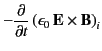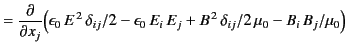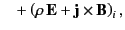(122)

because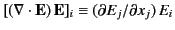, and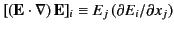. Here,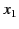corresponds to,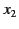to, and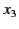to. Furthermore,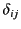is a Kronecker delta symbol (i.e.,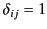if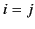, and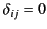otherwise). Finally, we are making use of the Einstein summation convention (that repeated indices are summed from 1 to 3). Comparing the previous expression with Equation (112), we conclude that the momentum density of electromagnetic fields takes the form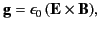(123)

whereas the corresponding momentum flux tensor has the Cartesian components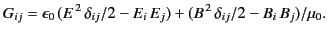(124)

The momentum conservation equation, (112), is sometimes written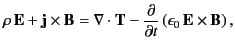(125)

where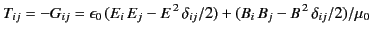(126)

is called the Maxwell stress tensor.Next: Exercises Up: Maxwell's Equations Previous: Electromagnetic Energy Conservation
Richard Fitzpatrick 2014-06-27